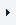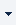LOGINREGISTER (FREE TRIAL)myTOMLAB
 ProductsTOMLAB Base ModuleTOMLAB /MINOSTOMLAB /NPSOLTOMLAB /DNOPTTOMLAB /SNOPTTOMLAB /SOLTOMLAB /CGOTOMLAB /CPLEXTOMLAB /GUROBITOMLAB /MINLPSolversTOMLAB /MIPNLPTOMLAB /MISQPTOMLAB /PENSDPTOMLAB /PENBMITOMLAB /KNITROTOMLAB /OQNLPTOMLAB /CONOPTTOMLAB /PROPTTOMLAB /NLPQLTOMLAB /LGOTOMLAB /LGO-MINLPTOMLAB /GPTOMLAB /GENOTOMLAB /MADTOMLAB /MIDACO

# TOMLAB /MINLP

TOMLAB /MINLP provides an advanced Matlab solution which includes four solvers developed by Roger Fletcher and Sven Leyffer at the University of Dundee. The solvers have been compiled in both a sparse and a dense version.

## Features and capabilities

• The solver MINLPbb solves large, sparse or dense mixed-integer linear, quadratic and nonlinear programming problems. MINLP implements a branch-and-bound algorithm searching a tree whose nodes correspond to continuous nonlinearly constrained optimization problems. The continuous problems are solved using filterSQP, see below.

• The filterSQP solver is a Sequential Quadratic Programming solver suitable for solving large, sparse or dense linear, quadratic and nonlinear programming problems. The method avoids the use of penalty functions. Global convergence is enforced through the use of a trust--region and the new concept of a filter which accepts a trial point whenever the objective or the constraint violation is improved compared to all previous iterates. The size of the trust--region is reduced if the step is rejected and increased if it is accepted, provided the agreement between the quadratic model and the nonlinear functions is sufficiently good.

• The solver MIQPbb solves sparse and dense mixed-integer linear and quadratic programs.

• The BQPD code solves quadratic programming (minimization of a quadratic function subject to linear constraints) and linear programming problems. If the Hessian matrix Q is positive definite, then a global solution is found. A global solution is also found in the case of linear programming (Q=0). When Q is indefinite, a Kuhn-Tucker point that is usually a local solution is found.

The code implements a null-space active set method with a technique for resolving degeneracy that guarantees that cycling does not occur even when round-off errors are present. Feasibility is obtained by minimizing a sum of constraint violations. The Devex method for avoiding near-zero pivots is used to promote stability. The matrix algebra is implemented so that the algorithm can take advantage of sparse factors of the basis matrix. Factors of the reduced Hessian matrix are stored in a dense format, an approach that is most effective when the number of free variables is relatively small. The user must supply a subroutine to evaluate the Hessian matrix Q, so that sparsity in Q can be exploited. An extreme case occurs when Q=0 and the QP reduces to a linear program. The code is written to take maximum advantage of this situation, so that it also provides an efficient method for linear programming.

• TOMLAB /MINLP is integrated with the TOMLAB optimization environment.

• The TOMLAB /MINLP solvers may be used as subproblem solvers in the TOMLAB environment.

## Requirements

• MATLAB 6.5 or later